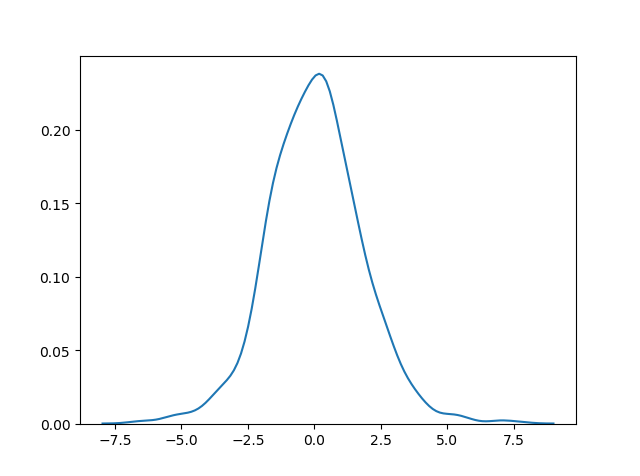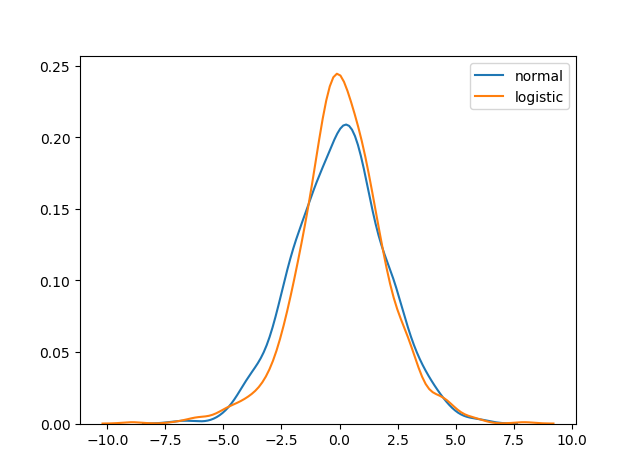# Logistic Distribution

## Logistic Distribution

Logistic Distribution is used to describe growth.

Used extensively in machine learning in logistic regression, neural networks etc.

It has three parameters:

`loc` - mean, where the peak is. Default 0.

`scale` - standard deviation, the flatness of distribution. Default 1.

`size` - The shape of the returned array.

### Example

Draw 2x3 samples from a logistic distribution with mean at 1 and stddev 2.0:

from numpy import random

x = random.logistic(loc=1, scale=2, size=(2, 3))

print(x)
Try it Yourself »

## Visualization of Logistic Distribution

### Example

from numpy import random
import matplotlib.pyplot as plt
import seaborn as sns

sns.distplot(random.logistic(size=1000), hist=False)

plt.show()

### ResultTry it Yourself »

## Difference Between Logistic and Normal Distribution

Both distributions are near identical, but logistic distribution has more area under the tails, meaning it represents more possibility of occurrence of an event further away from mean.

For higher value of scale (standard deviation) the normal and logistic distributions are near identical apart from the peak.

### Example

from numpy import random
import matplotlib.pyplot as plt
import seaborn as sns

sns.distplot(random.normal(scale=2, size=1000), hist=False, label='normal')
sns.distplot(random.logistic(size=1000), hist=False, label='logistic')

plt.show()

### ResultTry it Yourself »